# NCERT Solutions for Class 9 Maths Exercise 1.6## myCBSEguide App

CBSE, NCERT, JEE Main, NEET-UG, NDA, Exam Papers, Question Bank, NCERT Solutions, Exemplars, Revision Notes, Free Videos, MCQ Tests & more.

NCERT solutions for Class 9 Maths Number Systems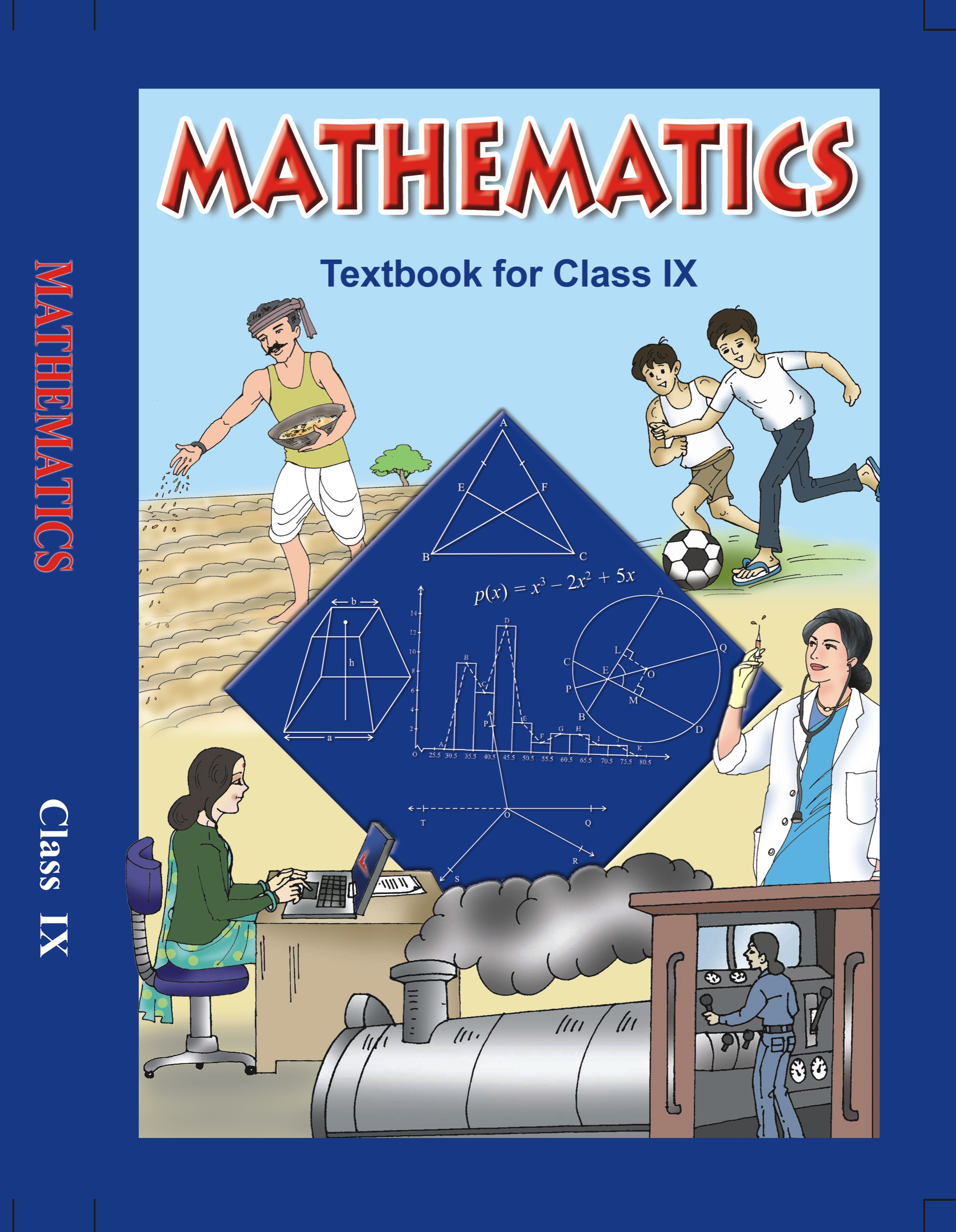## NCERT Solutions for Class 9 Maths Number Systems

###### 1. Find:(i)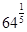(ii)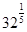(iii)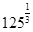Ans. (i)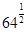We know that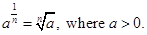We conclude thatcan also be written as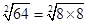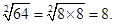Therefore, the value ofwill be 8.

###### (ii)We know thatWe conclude thatcan also be written as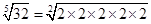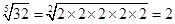Therefore, the value ofwill be 2.

(iii)We know thatWe conclude thatcan also be written as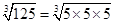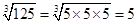Therefore, the value ofwill be 5.

NCERT Solutions for Class 9 Maths Exercise 1.6

###### 2. Find:(i)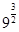(ii)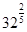(iii)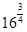(iv)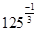Ans. (i)We know thatWe conclude thatcan also be written as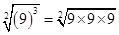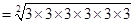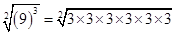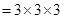=27

Therefore, the value ofwill be 27.

(ii)###### We know thatWe conclude thatcan also be written as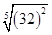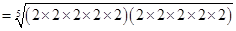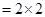= 4

Therefore, the value ofwill be 4.

(iii)We know thatWe conclude thatcan also be written as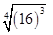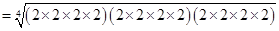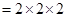= 8

###### Therefore, the value ofwill be 8.

(iv)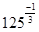We know that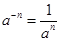We conclude thatcan also be written as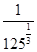, or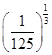.

We know thatWe know thatcan also be written as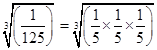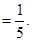Therefore, the value ofwill be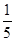.

NCERT Solutions for Class 9 Maths Exercise 1.6

###### 3. Simplify:

(i)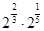(ii)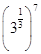(iii)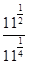(iv)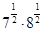Ans. (i)We know that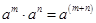.

We can conclude that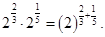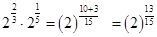Therefore, the value ofwill be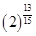.

(ii)We know that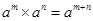###### We conclude thatcan also be written as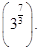.

(iii)We know that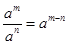We conclude that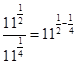.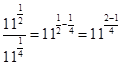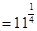Therefore, the value ofwill be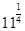.

(iv)We know that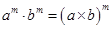.

We can conclude that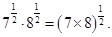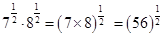Therefore, the value ofwill be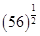.

## NCERT Solutions for Class 9 Maths Exercise 1.6

NCERT Solutions Class 9 Maths PDF (Download) Free from myCBSEguide app and myCBSEguide website. Ncert solution class 9 Maths includes text book solutions from Mathematics Book. NCERT Solutions for CBSE Class 9 Maths have total 15 chapters. 9 Maths NCERT Solutions in PDF for free Download on our website. Ncert Maths class 9 solutions PDF and Maths ncert class 9 PDF solutions with latest modifications and as per the latest CBSE syllabus are only available in myCBSEguide.

## CBSE app for Class 9

To download NCERT Solutions for Class 9 Maths, Computer Science, Home Science,Hindi ,English, Social Science do check myCBSEguide app or website. myCBSEguide provides sample papers with solution, test papers for chapter-wise practice, NCERT solutions, NCERT Exemplar solutions, quick revision notes for ready reference, CBSE guess papers and CBSE important question papers. Sample Paper all are made available through the best app for CBSE students and myCBSEguide website.### 11 thoughts on “NCERT Solutions for Class 9 Maths Exercise 1.6”

1. It good for 9standard

2. It good for 9standard

3. Goooood

4. Good for 9th class student

5. Very nice solutions